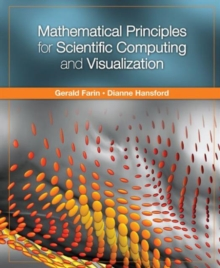Supporting your high street Find out how »
• My AccountMathematical Principles for Scientific Computing and Visualization Hardback

Description

This non-traditional introduction to the mathematics of scientific computation describes the principles behind the major methods, from statistics, applied mathematics, scientific visualization, and elsewhere, in a way that is accessible to a large part of the scientific community.

Introductory material includes computational basics, a review of coordinate systems, an introduction to facets (planes and triangle meshes) and an introduction to computer graphics.

The scientific computing part of the book covers topics in numerical linear algebra (basics, solving linear system, eigen-problems, SVD, and PCA) and numerical calculus (basics, data fitting, dynamic processes, root finding, and multivariate functions).

The visualization component of the book is separated into three parts: empirical data, scalar values over 2D data, and volumes.

Information

• Format: Hardback
• Pages: 280 pages
• Publisher: Taylor & Francis Inc
• Publication Date:
• Category: Applied mathematics
• ISBN: 9781568813219

£52.99

£47.25

on all orders

Pick up orders

from local bookshops

£63.99

£56.59

£45.99

£40.69